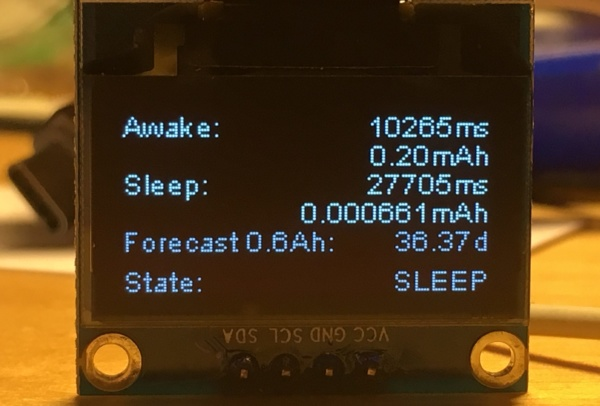# Arduino Motion Detection Simple Project

This is a very basic tutorial for Arduino beginners , we tried to keep it simple and to the point, how to use PIR motion detection sensors and how to control LED based on the sensor outpu.

and so simply put,The main idea of this project is to light a number of LEDs after sensing a motion with the PIR Sensor, LEDs are going to be turned on with a specific color which here we chose to be purple.. otherwise the LEDs are going to be off.

## Step 1: Step 1:What Will You Need

For this project we will need :

• LED
• PIR sensor
• Arduino
• Jumpers

## Step 2: How to Connect Them

1. Connect PIR Sensor to Arduino :
1. – Yellow wire to PIN 2 in the Arduino
2. – Black to GND
3. – Red to 5V
2. All LEDs to PIN 13 by using the breadboard as shown in the figure below

just follow the circuit diagram at the top, you can’t go wrong

## Step 3: Copy the Code

Just copy the code to Arduino IDE , and upolad it to your Arduino , all commands are commented , so you can read it line by line to understand the whole thing

Code:

int ledPin = 13; // choose the pin for the LED

int inputPin = 2; // choose the input pin (for PIR sensor)

int pirState = LOW; // we start, assuming no motion detected

int val = 0; // variable for reading the pin status

//variables needed to store values

long pulse, inches, cm;

void setup() {

pinMode(ledPin, OUTPUT); // declare LED as output

pinMode(inputPin, INPUT); // declare sensor as input

Serial.begin(9600);

}

void loop(){

if (val == HIGH) { // check if the input is HIGH

setColor(250,0,255,ledPin); // choose the color value

digitalWrite(ledPin, HIGH); // turn LED ON

if (pirState == LOW) {

// we have just turned on

Serial.println(“Motion detected!”);

// We only want to print on the output change, not state

pirState = HIGH;

pulse = pulseIn(inputPin, HIGH);

//147uS per inch

inches = pulse/147;

//change inches to centimetres

cm = inches * 2.54;

Serial.print(inches); // print for the inches value

Serial.print(“in, “);

Serial.print(cm);

Serial.print(“cm”); // print for the centinetres value

Serial.println();

delay(500);

}

} else {

digitalWrite(ledPin, LOW); // turn LED OFF

if (pirState == HIGH){

// we have just turned of

Serial.println(“Motion ended!”);

// We only want to print on the output change, not state

pirState = LOW;

}

}

}

void setColor(int red, int green, int blue,int led) // color function to set the color you want for the LED

{

#ifdef COMMON_ANODE

red = 255 – red; // the red channel value

green = 255 – green; // the green channel value

blue = 255 – blue; // the blue channel value

#endif

// dedicate every channel value to get one color

analogWrite(led, red);

analogWrite(led, green);

analogWrite(led, blue);

}

## Step 4: Conclusion

there’s nothing much to say , whenever you wave your hand near the sensor, you gonna see the LEDs blinking